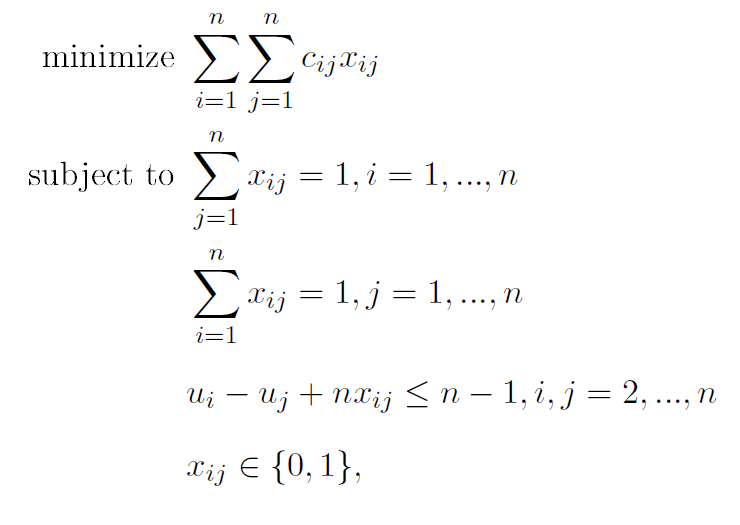# Multiobjective Multiple Traveling Salesman ProblemMy name is André Oliveira and I’m a student at the University of Coimbra, Portugal. I graduated with a degree in Mathematics and I’m currently taking my master’s degree in statistics, optimization and financial mathematics. During the first year of my masters I studied each of these fields, never having a clue about what I would like to study in my thesis, but my wish always was to make something with some strong applications. With lots of help and hard work, I ended up in a project in cooperation with the company Smartgeo. The company’s aim is to develop a computer application that estimates good vehicle routes in real time. This involves the development of algorithms regarding two well-known optimization problems: the Shortest Path Problem and the Traveling Salesman Problem (TSP).

My part of the project is the development and implementation of an algorithm that finds good solutions for the TSP considering multiple vehicles and optimizing several objectives simultaneously. This is known as the Multiobjective Multiple TSP and it has many applications like crew scheduling, school bus routing problems or even vehicle routing problems. To approach this problem, I started by studying the TSP and develop some new heuristics to solve it. Then I continued by studying the Multiple TSP (mTSP) and finally moved to the Multiobjective mTSP.The TSP is quite simple to state: given a set of cities that a traveling salesman must visit, find the best way to visit every city exactly once an then return to the original city, covering the shortest distance possible. The problem is usually represented in a graph G = (V, E) where the set of nodes/vertices V represents the cities and the set of arcs/edges E represents the paths between the cities. Each arc has a cost (or vector of costs) associated. The tour corresponding to the problem’s solution is the shortest Hamiltonian cycle of G. Despite the simplicity of its statement, the TSP is an NP-hard problem, which means that, unless P = NP, it can’t be solved in polynomial time.

The problem can be formulated as an integer linear problem:where xij is a binary variable that takes value 1 if the traveling salesman goes from i to j and 0 otherwise, cij is the cost from i to j and ui is an arbitrary number. The first two restrictions assure that each city is visited exactly once and the third assures that the solution has a single cycle and not a set of cycles that covers all the cities. We can solve the TSP using exact algorithms, using for example branch and bound. However, for large instances, it’s usual to use heuristics that produce good (usually not exact) solutions in less time.

The mTSP is similar to the TSP. The only difference is that we want to find a set of routes, one for each traveling salesman, instead of a single route. Each city must be visited by one and only one traveling salesman, one time. One interesting way to solve the mTSP is to transform it in a TSP and solve it using any TSP algorithm/heuristic. This is done generally by increasing the number of cities of the problem.

Currently, I’m dealing with the multiobjective part of the problem. The arc (i,j) has associated not a single cost cij but a vector of costs, that can represent distance, time, money cost, etc. Usually we have conflictive objectives, so the usual notion of optimal solution does not apply in this version of the problem. We say that a solution z dominates a solution w if, for every objective i, we have that zi ≤ wi and for some and at least one objective j, zj < wj. We solve the problem by finding the set of solutions that are not dominated by every other solution (Pareto solutions).

Until the end of the semester, I expect to finish the development of a heuristic that can find good solutions in real time for the multiobjective mTSP.

Best regards,

André Oliveira